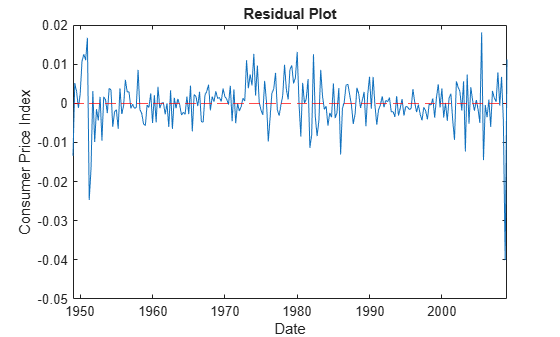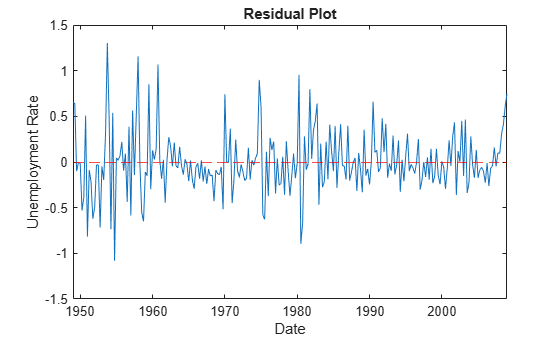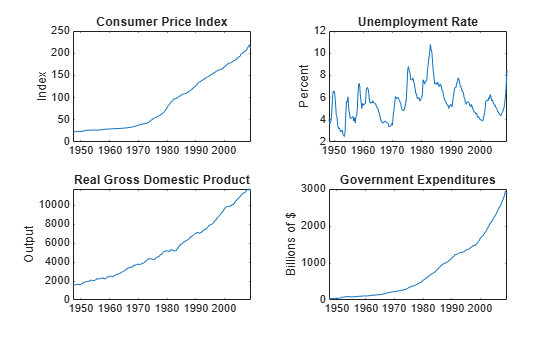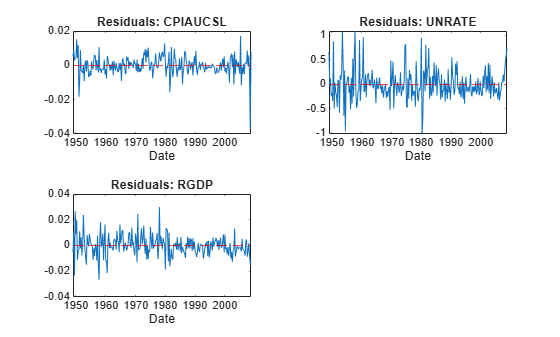# infer

Infer vector autoregression model (VAR) innovations

## Syntax

``E = infer(Mdl,Y)``
``Tbl2 = infer(Mdl,Tbl1)``
``___ = infer(___,Name,Value)``
``````[___,logL] = infer(___)``````

## Description

example

````E = infer(Mdl,Y)` returns a numeric array `E` containing the series of multivariate inferred innovations from evaluating the fully specified VAR(p) model `Mdl` at the numeric array of response data `Y`. For example, if `Mdl` is a VAR model fit to the response data `Y`, `E` contains the residuals.```

example

````Tbl2 = infer(Mdl,Tbl1)` returns the table or timetable `Tbl2` containing the multivariate residuals from evaluating the fully specified VAR(p) model `Mdl` at the response variables in the table or timetable of data `Tbl1`.```

example

````___ = infer(___,Name,Value)` specifies options using one or more name-value arguments in addition to any of the input argument combinations in previous syntaxes. `infer` returns the output argument combination for the corresponding input arguments. For example, `infer(Mdl,Y,Y0=PS,X=Exo)` computes the residuals of the VAR(p) model `Mdl` at the matrix of response data `Y`, and specifies the matrix of presample response data `PS` and the matrix of exogenous predictor data `Exo`.Supply all input data using the same data type. Specifically: If you specify the numeric matrix `Y`, optional data sets must be numeric arrays and you must use the appropriate name-value argument. For example, to specify a presample, set the `Y0` name-value argument to a numeric matrix of presample data.If you specify the table or timetable `Tbl1`, optional data sets must be tables or timetables, respectively, and you must use the appropriate name-value argument. For example, to specify a presample, set the `Presample` name-value argument to a table or timetable of presample data. ```

example

``````[___,logL] = infer(___)``` returns the loglikelihood objective function value `logL` evaluated at the specified data.```

## Examples

collapse all

Fit a VAR(4) model to the consumer price index (CPI) and unemployment rate data in a matrix. Then, infer the model innovations (residuals) from the estimated model.

Load the `Data_USEconModel` data set.

`load Data_USEconModel`

Plot the two series on separate plots.

```figure plot(DataTimeTable.Time,DataTimeTable.CPIAUCSL) title("Consumer Price Index") ylabel("Index") xlabel("Date")``````figure plot(DataTimeTable.Time,DataTimeTable.UNRATE) title("Unemployment Rate") ylabel("Percent") xlabel("Date")```Stabilize the CPI by converting it to a series of growth rates. Synchronize the two series by removing the first observation from the unemployment rate series.

```rcpi = price2ret(DataTimeTable.CPIAUCSL); unrate = DataTimeTable.UNRATE(2:end);```

Create a default VAR(4) model using the shorthand syntax.

`Mdl = varm(2,4);`

Estimate the model using the entire data set.

`EstMdl = estimate(Mdl,[rcpi unrate]);`

`EstMdl` is a fully specified, estimated `varm` model object.

Infer innovations from the estimated model. Supply the same response data that the model was fit to as a numeric matrix.

`E = infer(EstMdl,[rcpi unrate]);`

`E` is a 241-by-2 matrix of inferred innovations. The first and second columns contain the residuals corresponding to the CPI growth rate and unemployment rate, respectively.

Alternatively, you can return residuals when you call `estimate` by supplying an output variable in the fourth position.

Plot the residuals on separate plots. Synchronize the residuals with the dates by removing any missing observations from the data and removing the first `Mdl.P` dates.

```idx = all(~isnan([rcpi unrate]),2); datesr = DataTimeTable.Time(idx); figure plot(datesr((Mdl.P + 1):end),E(:,1)); ylabel("Consumer Price Index") xlabel("Date") title("Residual Plot") hold on yline(0,"r--"); hold off``````figure plot(datesr((Mdl.P + 1):end),E(:,2)) ylabel("Unemployment Rate") xlabel("Date") title("Residual Plot") hold on yline(0,"r--"); hold off```The residuals corresponding to the CPI growth rate exhibit heteroscedasticity because the series appears to cycle through periods of higher and lower variance.

Fit a VAR(4) model to the consumer price index (CPI) and unemployment rate data in a timetable. Then, infer the model innovations (residuals) from the estimated model.

Load the `Data_USEconModel` data set. Compute the CPI growth rate. Because the growth rate calculation consumes the earliest observation, include the rate variable in the timetable by prepending the series with `NaN`.

```load Data_USEconModel DataTimeTable.RCPI = [NaN; price2ret(DataTimeTable.CPIAUCSL)]; numobs = height(DataTimeTable)```
```numobs = 249 ```

Prepare Timetable for Estimation

When you plan to supply a timetable directly to estimate, you must ensure it has all the following characteristics:

• All selected response variables are numeric and do not contain any missing values.

• The timestamps in the `Time` variable are regular, and they are ascending or descending.

Remove all missing values from the table, relative to the CPI rate (`RCPI`) and unemployment rate (`UNRATE`) series.

```varnames = ["RCPI" "UNRATE"]; DTT = rmmissing(DataTimeTable,DataVariables=varnames); numobs = height(DTT)```
```numobs = 245 ```

`rmmissing` removes the four initial missing observations from the `DataTimeTable` to create a sub-table `DTT`. The variables `RCPI` and `UNRATE` of `DTT` do not have any missing observations.

Determine whether the sampling timestamps have a regular frequency and are sorted.

`areTimestampsRegular = isregular(DTT,"quarters")`
```areTimestampsRegular = logical 0 ```
`areTimestampsSorted = issorted(DTT.Time)`
```areTimestampsSorted = logical 1 ```

`areTimestampsRegular = 0` indicates that the timestamps of `DTT` are irregular. `areTimestampsSorted = 1` indicates that the timestamps are sorted. Macroeconomic series in this example are timestamped at the end of the month. This quality induces an irregularly measured series.

Remedy the time irregularity by shifting all dates to the first day of the quarter.

```dt = DTT.Time; dt = dateshift(dt,"start","quarter"); DTT.Time = dt; areTimestampsRegular = isregular(DTT,"quarters")```
```areTimestampsRegular = logical 1 ```

`DTT` is regular with respect to time.

Create Model Template for Estimation

Create a default VAR(4) model using the shorthand syntax. Specify the response variable names.

```Mdl = varm(2,4); Mdl.SeriesNames = varnames;```

Fit Model to Data

Estimate the model. Pass the entire timetable `DTT`. By default, `estimate` selects the response variables in `Mdl.SeriesNames` to fit to the model. Alternatively, you can use the `ResponseVariables` name-value argument.

`EstMdl = estimate(Mdl,DTT);`

Compute Residuals

Infer innovations from the estimated model. Supply the same response data that the model was fit to as a timetable. By default, `infer` selects the variables to use from `EstMdl.SeriesNames`.

```Tbl = infer(EstMdl,DTT); head(Tbl)```
``` Time COE CPIAUCSL FEDFUNDS GCE GDP GDPDEF GPDI GS10 HOANBS M1SL M2SL PCEC TB3MS UNRATE RCPI RCPI_Residuals UNRATE_Residuals _____ _____ ________ ________ ____ _____ ______ ____ ____ ______ ____ ____ _____ _____ ______ __________ ______________ ________________ Q1-49 144.1 23.91 NaN 45.6 270 16.531 40.9 NaN 53.961 NaN NaN 177 1.17 5 -0.0058382 -0.013422 0.64674 Q2-49 141.9 23.92 NaN 47.3 266.2 16.35 34 NaN 53.058 NaN NaN 178.6 1.17 6.2 0.00041815 0.0051673 0.6439 Q3-49 141 23.75 NaN 47.2 267.7 16.256 37.3 NaN 52.501 NaN NaN 178 1.07 6.6 -0.0071324 0.0030175 -0.099092 Q4-49 140.5 23.61 NaN 46.6 265.2 16.272 35.2 NaN 52.291 NaN NaN 180.4 1.1 6.6 -0.0059122 -0.001196 -0.0066535 Q1-50 144.6 23.64 NaN 45.6 275.2 16.222 44.4 NaN 52.696 NaN NaN 183.1 1.12 6.3 0.0012698 0.0024607 -0.013354 Q2-50 150.6 23.88 NaN 46.1 284.6 16.286 49.9 NaN 53.997 NaN NaN 187 1.15 5.4 0.010101 0.010823 -0.53098 Q3-50 159 24.34 NaN 45.9 302 16.63 56.1 NaN 55.7 NaN NaN 200.7 1.3 4.4 0.01908 0.012566 -0.38177 Q4-50 166.9 24.98 NaN 49.5 313.4 16.95 65.9 NaN 56.213 NaN NaN 198.1 1.34 4.3 0.025954 0.010998 0.50761 ```
`size(Tbl)`
```ans = 1×2 241 17 ```

`Tbl` is a 241-by-17 timetable of variables in `DTT` and estimated model residuals, `RCPI_Residuals` and `UNRATE_Residuals`.

Alternatively, you can return residuals when you call `estimate` by supplying an output variable in the fourth position.

Estimate a VAR(4) model of the consumer price index (CPI), the unemployment rate, and the gross domestic product (GDP). Include a linear regression component containing the current quarter and the last four quarters of government consumption expenditures and investment (GCE). Infer model innovations.

Load the `Data_USEconModel` data set. Compute the real GDP.

```load Data_USEconModel DataTimeTable.RGDP = DataTimeTable.GDP./DataTimeTable.GDPDEF*100;```

Plot all variables on separate plots.

```figure tiledlayout(2,2) nexttile plot(DataTimeTable.Time,DataTimeTable.CPIAUCSL); ylabel("Index") title("Consumer Price Index") nexttile plot(DataTimeTable.Time,DataTimeTable.UNRATE); ylabel("Percent") title("Unemployment Rate") nexttile plot(DataTimeTable.Time,DataTimeTable.RGDP); ylabel("Output") title("Real Gross Domestic Product") nexttile plot(DataTimeTable.Time,DataTimeTable.GCE); ylabel("Billions of \$") title("Government Expenditures")```Stabilize the CPI, GDP, and GCE by converting each to a series of growth rates. Synchronize the unemployment rate series with the others by removing its first observation.

```varnames = ["CPIAUCSL" "RGDP" "GCE"]; DTT = varfun(@price2ret,DataTimeTable,InputVariables=varnames); DTT.Properties.VariableNames = varnames; DTT.UNRATE = DataTimeTable.UNRATE(2:end);```

Make the time base regular.

```dt = DTT.Time; dt = dateshift(dt,"start","quarter"); DTT.Time = dt;```

Expand the GCE rate series to a matrix that includes the first lagged series through the fourth lag series.

```RGCELags = lagmatrix(DTT,1:4,DataVariables="GCE"); DTT = [DTT RGCELags]; DTT = rmmissing(DTT);```

Create a default VAR(4) model using the shorthand syntax. Specify the response variable names.

```Mdl = varm(3,4); Mdl.SeriesNames = ["CPIAUCSL" "UNRATE" "RGDP"];```

Estimate the model using the entire sample. Specify the GCE and its lags as exogenous predictor data for the regression component.

```prednames = contains(DTT.Properties.VariableNames,"GCE"); EstMdl = estimate(Mdl,DTT,PredictorVariables=prednames);```

Infer innovations from the estimated model. Supply the predictor data. Return the loglikelihood objective function value.

```[Tbl,logL] = infer(EstMdl,DTT,PredictorVariables=prednames); size(Tbl)```
```ans = 1×2 240 11 ```
`head(Tbl)`
``` Time CPIAUCSL RGDP GCE UNRATE Lag1GCE Lag2GCE Lag3GCE Lag4GCE CPIAUCSL_Residuals UNRATE_Residuals RGDP_Residuals _____ __________ __________ __________ ______ __________ __________ __________ __________ __________________ ________________ ______________ Q1-49 0.00041815 -0.0031645 0.036603 6.2 0.047147 0.04948 0.04193 0.054347 0.0053457 0.6564 -0.0053201 Q2-49 -0.0071324 0.011385 -0.0021164 6.6 0.036603 0.047147 0.04948 0.04193 0.0088626 -0.034796 0.010153 Q3-49 -0.0059122 -0.010366 -0.012793 6.6 -0.0021164 0.036603 0.047147 0.04948 0.0029402 0.11695 -0.02318 Q4-49 0.0012698 0.040091 -0.021693 6.3 -0.012793 -0.0021164 0.036603 0.047147 0.0040774 -0.2343 0.026583 Q1-50 0.010101 0.029649 0.010905 5.4 -0.021693 -0.012793 -0.0021164 0.036603 0.0046233 -0.18043 0.0091538 Q2-50 0.01908 0.03844 -0.0043478 4.4 0.010905 -0.021693 -0.012793 -0.0021164 0.015141 -0.34049 0.019797 Q3-50 0.025954 0.017994 0.075508 4.3 -0.0043478 0.010905 -0.021693 -0.012793 0.0041785 0.87368 -0.011263 Q4-50 0.035395 0.01197 0.14807 3.4 0.075508 -0.0043478 0.010905 -0.021693 0.011772 -0.49694 -0.0044563 ```
`logL`
```logL = 1.7056e+03 ```

`Tbl` is a 240-by-11 timetable of data and inferred innovations from the estimated model (residuals).

Plot the residuals on separate plots.

```idx = endsWith(Tbl.Properties.VariableNames,"_Residuals"); resvars = Tbl.Properties.VariableNames(idx); titles = "Residuals: " + EstMdl.SeriesNames; figure tiledlayout(2,2) for j = 1:Mdl.NumSeries nexttile plot(Tbl.Time,Tbl{:,resvars(j)}); xlabel("Date"); title(titles(j)); hold on yline(0,"r--"); hold off end```The residuals corresponding to the CPI and GDP growth rates exhibit heteroscedasticity because the CPI series appears to cycle through periods of higher and lower variance. Also, the first half of the GDP series seems to have higher variance than the latter half.

## Input Arguments

collapse all

VAR model, specified as a `varm` model object created by `varm` or `estimate`. `Mdl` must be fully specified.

Response data, specified as a `numobs`-by-`numseries` numeric matrix or a `numobs`-by-`numseries`-by-`numpaths` numeric array.

`numobs` is the sample size. `numseries` is the number of response series (`Mdl.NumSeries`). `numpaths` is the number of response paths.

Rows correspond to observations, and the last row contains the latest observation. `Y` represents the continuation of the presample response series in `Y0`.

Columns must correspond to the response variable names in `Mdl.SeriesNames`.

Pages correspond to separate, independent `numseries`-dimensional paths. Among all pages, responses in a particular row occur at the same time.

Data Types: `double`

Time series data containing observed response variables yt and, optionally, predictor variables xt for a model with a regression component, specified as a table or timetable with `numvars` variables and `numobs` rows.

Each selected response variable is a `numobs`-by-`numpaths` numeric matrix, and each selected predictor variable is a numeric vector. Each row is an observation, and measurements in each row occur simultaneously. You can optionally specify `numseries` response variables by using the `ResponseVariables` name-value argument, and you can specify `numpreds` predictor variables by using the `PredictorVariables` name-value argument.

Paths (columns) within a particular response variable are independent, but path `j` of all variables correspond, for `j` = 1,…,`numpaths`.

If `Tbl1` is a timetable, it must represent a sample with a regular datetime time step (see `isregular`), and the datetime vector `Tbl1.Time` must be strictly ascending or descending.

If `Tbl1` is a table, the following conditions hold:

• The last row contains the latest observation.

• `Tbl1.Properties.RowsNames` must be empty.

### Name-Value Arguments

Specify optional pairs of arguments as `Name1=Value1,...,NameN=ValueN`, where `Name` is the argument name and `Value` is the corresponding value. Name-value arguments must appear after other arguments, but the order of the pairs does not matter.

Before R2021a, use commas to separate each name and value, and enclose `Name` in quotes.

Example: `infer(Mdl,Y,Y0=PS,X=Exo)` computes the residuals of the VAR(p) model `Mdl` at the matrix of response data `Y`, and specifies the matrix of presample response data `PS` and the matrix of exogenous predictor data `Exo`.

Variables to select from `Tbl1` to treat as response variables yt, specified as one of the following data types:

• String vector or cell vector of character vectors containing `numseries` variable names in `Tbl1.Properties.VariableNames`

• A length `numseries` vector of unique indices (integers) of variables to select from `Tbl1.Properties.VariableNames`

• A length `numvars` logical vector, where ```ResponseVariables(j) = true``` selects variable `j` from `Tbl1.Properties.VariableNames`, and `sum(ResponseVariables)` is `numseries`

The selected variables must be numeric vectors (single path) or matrices (columns represent multiple independent paths) of the same width, and cannot contain missing values (`NaN`).

If the number of variables in `Tbl1` matches `Mdl.NumSeries`, the default specifies all variables in `Tbl1`. If the number of variables in `Tbl1` exceeds `Mdl.NumSeries`, the default matches variables in `Tbl1` to names in `Mdl.SeriesNames`.

Example: `ResponseVariables=["GDP" "CPI"]`

Example: `ResponseVariables=[true false true false]` or `ResponseVariable=[1 3]` selects the first and third table variables as the response variables.

Data Types: `double` | `logical` | `char` | `cell` | `string`

Presample responses that provide initial values for the model `Mdl`, specified as a `numpreobs`-by-`numseries` numeric matrix or a `numpreobs`-by-`numseries`-by-`numprepaths` numeric array. Use `Y0` only when you supply a numeric array of response data `Y`.

`numpreobs` is the number of presample observations. `numprepaths` is the number of presample response paths.

Each row is a presample observation, and measurements in each row, among all pages, occur simultaneously. The last row contains the latest presample observation. `Y0` must have at least `Mdl.P` rows. If you supply more rows than necessary, `infer` uses the latest `Mdl.P` observations only.

Each column corresponds to the response series associated with the respective response series in `Y`.

Pages correspond to separate, independent paths.

• If `Y0` is a matrix, `infer` applies it to each path (page) in `Y`. Therefore, all paths in `Y` derive from common initial conditions.

• Otherwise, `infer` applies `Y0(:,:,j)` to `Y(:,:,j)`. `Y0` must have at least `numpaths` pages, and `infer` uses only the first `numpaths` pages.

By default, `infer` uses the first `Mdl.P` observations, for example, `Y(1:Mdl.P,:)`, as a presample. This action reduces the effective sample size.

Data Types: `double`

Presample data that provide initial values for the model `Mdl`, specified as a table or timetable, the same type as `Tbl1`, with `numprevars` variables and `numpreobs` rows.

Each row is a presample observation, and measurements in each row, among all paths, occur simultaneously. `numpreobs` must be at least `Mdl.P`. If you supply more rows than necessary, `infer` uses the latest `Mdl.P` observations only.

Each variable is a `numpreobs`-by-`numprepaths` numeric matrix. Variables correspond to the response series associated with the respective response variable in `Tbl1`. To control presample variable selection, see the optional `PresampleResponseVariables` name-value argument.

For each variable, columns are separate, independent paths.

• If variables are vectors, `infer` applies them to each path in `Tbl1` to produce the corresponding residuals in `Tbl2`. Therefore, all response paths derive from common initial conditions.

• Otherwise, for each variable `ResponseK` and each path `j`, `infer` applies `Presample.ResponseK(:,j)` to produce `Tbl2.ResponseK(:,j)`. Variables must have at least `numpaths` columns, and `infer` uses only the first `numpaths` columns.

If `Presample` is a timetable, all the following conditions must be true:

• `Presample` must represent a sample with a regular datetime time step (see `isregular`).

• The inputs `Tbl1` and `Presample` must be consistent in time such that `Presample` immediately precedes `Tbl1` with respect to the sampling frequency and order.

• The datetime vector of sample timestamps `Presample.Time` must be ascending or descending.

If `Presample` is a table, the following conditions hold:

• The last row contains the latest presample observation.

• `Presample.Properties.RowsNames` must be empty.

By default, `infer` uses the first or earliest `Mdl.P` observations in `Tbl1` as a presample, and then it fits the model to the remaining `numobs - Mdl.P` observations. This action reduces the effective sample size.

Variables to select from `Presample` to use for presample data, specified as one of the following data types:

• String vector or cell vector of character vectors containing `numseries` variable names in `Presample.Properties.VariableNames`

• A length `numseries` vector of unique indices (integers) of variables to select from `Presample.Properties.VariableNames`

• A length `numvars` logical vector, where `PresampleResponseVariables(j) = true ` selects variable `j` from `Presample.Properties.VariableNames`, and `sum(PresampleResponseVariables)` is `numseries`

The selected variables must be numeric vectors (single path) or matrices (columns represent multiple independent paths) of the same width, and cannot contain missing values (`NaN`).

`PresampleResponseNames` does not need to contain the same names as in `Tbl1`; `infer` uses the data in selected variable `PresampleResponseVariables(j)` as a presample for the response variable corresponding to `ResponseVariables(j)`.

The default specifies the same response variables as those selected from `Tbl1`, see `ResponseVariables`.

Example: `PresampleResponseVariables=["GDP" "CPI"]`

Example: `PresampleResponseVariables=[true false true false]` or `PresampleResponseVariable=[1 3]` selects the first and third table variables for presample data.

Data Types: `double` | `logical` | `char` | `cell` | `string`

Predictor data xt for the regression component in the model, specified as a numeric matrix containing `numpreds` columns. Use `X` only when you supply a numeric array of response data `Y`.

`numpreds` is the number of predictor variables (`size(Mdl.Beta,2)`).

Each row corresponds to an observation, and measurements in each row occur simultaneously. The last row contains the latest observation. `X` must have at least as many observations as `Y`. If you supply more rows than necessary, `infer` uses only the latest observations. `infer` does not use the regression component in the presample period.

• If you specify a numeric array for a presample by using `Y0`, `X` must have at least `numobs` rows (see `Y`).

• Otherwise, `X` must have at least `numobs``Mdl.P` observations to account for the default presample removal from `Y`.

Each column is an individual predictor variable. All predictor variables are present in the regression component of each response equation.

`infer` applies `X` to each path (page) in `Y`; that is, `X` represents one path of observed predictors.

By default, `infer` excludes the regression component, regardless of its presence in `Mdl`.

Data Types: `double`

Variables to select from `Tbl1` to treat as exogenous predictor variables xt, specified as one of the following data types:

• String vector or cell vector of character vectors containing `numpreds` variable names in `Tbl1.Properties.VariableNames`

• A length `numpreds` vector of unique indices (integers) of variables to select from `Tbl1.Properties.VariableNames`

• A length `numvars` logical vector, where `PredictorVariables(j) = true ` selects variable `j` from `Tbl1.Properties.VariableNames`, and `sum(PredictorVariables)` is `numpreds`

The selected variables must be numeric vectors and cannot contain missing values (`NaN`).

By default, `infer` excludes the regression component, regardless of its presence in `Mdl`.

Example: `PredictorVariables=["M1SL" "TB3MS" "UNRATE"]`

Example: `PredictorVariables=[true false true false]` or `PredictorVariable=[1 3]` selects the first and third table variables as the response variables.

Data Types: `double` | `logical` | `char` | `cell` | `string`

Note

• `NaN` values in `Y`, `Y0`, and `X` indicate missing values. `infer` removes missing values from the data by list-wise deletion.

1. If `Y` is a 3-D array, then `infer` horizontally concatenates the pages of `Y` to form a `numobs`-by-```(numpaths*numseries + numpreds)``` matrix.

2. If a regression component is present, then `infer` horizontally concatenates `X` to `Y` to form a `numobs`-by-```numpaths*numseries + 1``` matrix. `infer` assumes that the last rows of each series occur at the same time.

3. `infer` removes any row that contains at least one `NaN` from the concatenated data.

4. `infer` applies steps 1 and 3 to the presample paths in `Y0`.

This process ensures that the inferred output innovations of each path are the same size and are based on the same observation times. In the case of missing observations, the results obtained from multiple paths of `Y` can differ from the results obtained from each path individually.

This type of data reduction reduces the effective sample size.

• `infer` issues an error when any table or timetable input contains missing values.

## Output Arguments

collapse all

Inferred multivariate innovations series, returned as either a numeric matrix, or as a numeric array that contains columns and pages corresponding to `Y`. `infer` returns `E` only when you supply a matrix of response data `Y`.

• If you specify `Y0`, then `E` has `numobs` rows (see `Y`).

• Otherwise, `E` has `numobs``Mdl.P` rows to account for the presample removal.

Inferred multivariate innovations series and other variables, returned as a table or timetable, the same data type as `Tbl1`. `infer` returns `Tbl2` only when you supply the input `Tbl1`.

`Tbl2` contains the inferred innovation paths `E` from evaluating the model `Mdl` at the paths of selected response variables `Y`, and it contains all variables in `Tbl1`. `infer` names the innovation variable corresponding to variable `ResponseJ` in `Tbl1` `ResponseJ_Residuals`. For example, if one of the selected response variables for estimation in `Tbl1` is `GDP`, `Tbl2` contains a variable for the residuals in the response equation of `GDP` with the name `GDP_Residuals`.

If you specify presample response data, `Tbl2` and `Tbl1` have the same number of rows, and their rows correspond. Otherwise, because `infer` removes initial observations from `Tbl1` for the required presample by default, `Tbl2` has `numobs - Mdl.P` rows to account for that removal.

If `Tbl1` is a timetable, `Tbl1` and `Tbl2` have the same row order, either ascending or descending.

Loglikelihood objective function value, returned as a numeric scalar or a `numpaths`-element numeric vector. `logL(j)` corresponds to the response path in `Y(:,:,j)` or the path (column) `j` of the selected response variables of `Tbl1`.

## Algorithms

Suppose `Y`, `Y0`, and `X` are the response, presample response, and predictor data specified by the numeric data inputs in `Y`, `Y0`, and `X`, or the selected variables from the input tables or timetables `Tbl1` and `Presample`.

• `infer` infers innovations by evaluating the VAR model `Mdl`, specifically

`${\stackrel{^}{\epsilon }}_{t}=\stackrel{^}{\Phi }\left(L\right){y}_{t}-\stackrel{^}{c}-\stackrel{^}{\beta }{x}_{t}-\stackrel{^}{\delta }t.$`

• `infer` uses this process to determine the time origin t0 of models that include linear time trends.

• If you do not specify `Y0`, then t0 = 0.

• Otherwise, `infer` sets t0 to `size(Y0,1)``Mdl.P`. Therefore, the times in the trend component are t = t0 + 1, t0 + 2,..., t0 + `numobs`, where `numobs` is the effective sample size (`size(Y,1)` after `infer` removes missing values). This convention is consistent with the default behavior of model estimation in which `estimate` removes the first `Mdl.P` responses, reducing the effective sample size. Although `infer` explicitly uses the first `Mdl.P` presample responses in `Y0` to initialize the model, the total number of observations in `Y0` and `Y` (excluding missing values) determines t0.

 Hamilton, James D. Time Series Analysis. Princeton, NJ: Princeton University Press, 1994.

 Johansen, S. Likelihood-Based Inference in Cointegrated Vector Autoregressive Models. Oxford: Oxford University Press, 1995.

 Juselius, K. The Cointegrated VAR Model. Oxford: Oxford University Press, 2006.

 Lütkepohl, H. New Introduction to Multiple Time Series Analysis. Berlin: Springer, 2005.